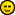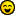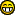cancel
Showing results for
Did you mean:

Highlighted

## Generate random number in Soap UI using Java

Hello,
I want to genere a random number inside an xml page opened in SoapUI, in order to test a web service.

I have tried this :
`<msisdn>079999\${=(int)(Math.random()*9999)} </msisdn>`

It works, but i need only 10 number digit (a phone number)

I thought I could use some java methods to get this :
`NumberFormat nf = NumberFormat.getInstance();nf.setMinimumIntegerDigits(4);nf.setGroupingUsed(false);System.out.println(nf.format((int)(Math.random()*9999)));`

Or :
`System.out.println(String.format("%04d", new Random().nextInt(9999)));Unfortunatly it does'nt work, because Groovy doesn't know NumberFormat or Radom...Have you any solution for my problem?Thanks for your help`
5 REPLIES 5
Highlighted

## Re: Generate random number in Soap UI using Java

Hi,

In a project I created with soapUI it was looking for a possibility to bring functionality to library. In the Open Source version of soapUI you create jars and you have to store those in folder <installation directory>/bin/ext/.

Maybe you can use this either. You just code you function in Java and create a jar-file. This can be used by Groovy script very easily.

To ensure a special number of digits I would recommend to use datatype String. In XML-document you will not have trouble with it.

This solution might not be the most efficient but it will work.

Best Regards
Dirk
Highlighted

## Re: Generate random number in Soap UI using Java

Hi,

If you want to create a random number with 10 digits, use the following groovy script:
`int a = 9nr = ""for(i = 0; i < 10; i++){ random = new Random() randomInteger= random.nextInt(a) nr = nr + randomInteger}log.info nr`

If you want to limit the value of a digit on a specific spot (for instance, the first digit is always a zero) you can use nr.lenght() to assert the digit-position:
`int a = 9nr = ""for(i = 0; i < 10; i++){ if(nr.length() < 1) {  nr = nr + "0" } else {  random = new Random()  randomInteger= random.nextInt(a)  nr = nr + randomInteger }}log.info nr`

Of course you still need to set the value of nr to the correct parameter in your XML...
Highlighted

## Re: Generate random number in Soap UI using Java

Thanks for your help, it works better than an other solution I have found later :
`<msisdn>079999\${=((int)Math.random()*(9999-1000)+1000)}</msisdn>`

The post is solved.
Highlighted

## Re: Generate random number in Soap UI using Java

This solution works great. I set the random number to stop at 5 digits:
int a = 9
nr = ""
for(i = 0; i < 5; i++)
{
random = new Random()
randomInteger= random.nextInt(a)
nr = nr + randomInteger
}
log.info nr

However, how would I make it so that the number generated couldn't go higher than say 50000?
Highlighted

## Re: Generate random number in Soap UI using Java

Hiya

Don't know if it would help but I am using a much shorter random number string that will write to a property.

//Generate TransactionID
testRunner.testCase.setPropertyValue("TransID", String.valueOf((int)Math.random()*1000000000) );

This gives me a random 9 digit number between 000000001 & 999999999.

DiNew Here?